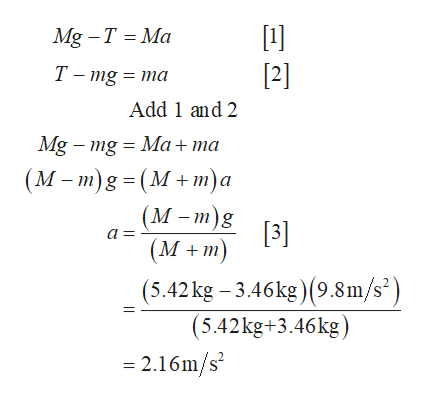On one end of a pulley an object M = 5.42 kg and another object with a m = 3.46 kg. Assume the pulley and the rope to be massless, and ignore all friction effects. a) Find the acceleration of the system.b) Find the tension in the rope connecting m & M.

Question

On one end of a pulley an object M = 5.42 kg and another object with a m = 3.46 kg. Assume the pulley and the rope to be massless, and ignore all friction effects.
a) Find the acceleration of the system.
b) Find the tension in the rope connecting m & M.

Step 1

The below represents the free body diagram of the pulley having mass on the either sides.

Step 2

(a)  Write the expression for net f...help_outlineImage Transcriptionclose1]  Mg T Ma Т-mg %3D тa Add 1 and 2 Mg mg = Ma+ma (М -т)g - (М+т)а (М-т)g  а - (M +m) (5.42kg 3.46kg) (9.8m/s2 (5.42kg+3.46kg) - 2.16m/s2 fullscreen

Want to see the full answer?

See Solution

Want to see this answer and more?

Our solutions are written by experts, many with advanced degrees, and available 24/7

See Solution
Tagged in

Newtons Laws of Motion